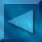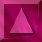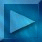Previous : Choice of the estimator  Next : Log-Likelihood derivatives Notations

 Calculus of the Likelihood

The different probability laws :

• Y follows a Gaussian distribution N (),  N=Y-HX :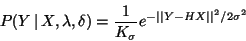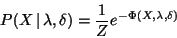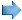The likelihood can be rewritten in the form :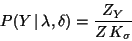Z, ZY = partition functions (cf. statistical mechanics) ,
they are normalizing constants.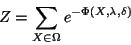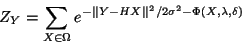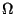is the set of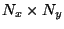images with M grey levels.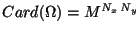, at least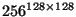: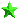Impossible to directly calculate the partition functions !
because of the dimension of thespace.We do not try to calculate them, but to estimate them.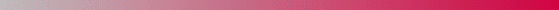André Jalobeanu - 24 Aug  1998